Function Repository Resource:

# RandomUnimodularMatrix

Return a pseudorandom unimodular matrix

Contributed by: Jan Mangaldan
 ResourceFunction["RandomUnimodularMatrix"][n] gives a pseudorandom n×n unimodular matrix.

## Details and Options

A unimodular matrix is a square integer matrix whose inverse also has integer entries.
Such a matrix has a determinant that is a unit (that is, 1 or -1 if not allowing Gaussian integers).
ResourceFunction["RandomUnimodularMatrix"] accepts the following options:
 GaussianIntegers False whether the matrix returned should have Gaussian integer entries "MaxEntry" Automatic bound on the matrix elements MaxIterations Automatic maximum number of iterations to use
With "MaxEntry"m, the absolute values of the entries of the matrix are guaranteed to be less than or equal to m.

## Examples

### Basic Examples (2)

Generate a random 5×5 unimodular matrix:

 In:=Out=Its inverse has integer entries:

 In:=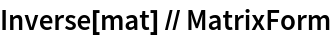Out=### Scope (1)

A medium-sized unimodular matrix:

 In:=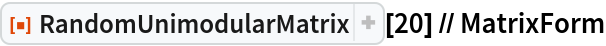Out=### Options (4)

#### GaussianIntegers (2)

Generate a unimodular matrix with Gaussian integer entries:

 In:=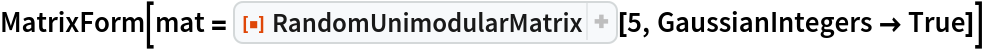Out=Its inverse has Gaussian integer entries:

 In:=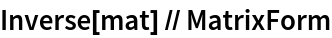Out=#### MaxEntry (1)

Generate a unimodular matrix with elements between -2 and 2:

 In:=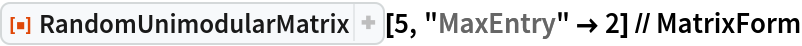Out=#### MaxIterations (1)

Change the number of iterations needed to generate the matrix:

 In:=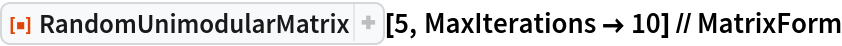Out=### Applications (2)

Generate an integer matrix with integer eigenvalues:

 In:=Out=Show its eigenvalues and eigenvectors:

 In:=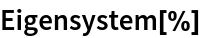Out=## Version History

• 1.0.0 – 03 March 2021